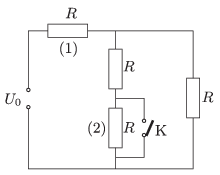Mathematical and Physical Journal
for High Schools
Issued by the MATFUND Foundation
 Already signed up? New to KöMaL?

#Problem P. 4764. (October 2015)

P. 4764. The resistance of each resistor shown in the figure is the same $\displaystyle R$. By what percent will the dissipated power at resistor (1) change if switch K is turned on to create a short circuit through the resistor (2)?(4 pont)

Deadline expired on November 10, 2015.

### Statistics:

 141 students sent a solution. 4 points: 95 students. 3 points: 21 students. 2 points: 15 students. 1 point: 5 students. 0 point: 3 students. Unfair, not evaluated: 2 solutionss.

Problems in Physics of KöMaL, October 2015